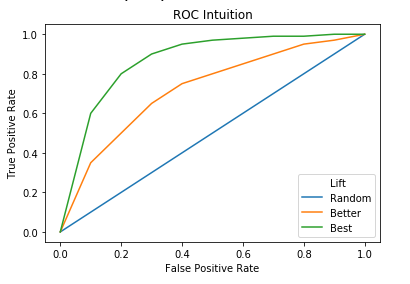# How would you evaluate a Classification model using ROC/AUC?

Another important concept in classification is the Receiver Operating Curve (ROC) and the corresponding area under the curve, or AUC. Unlike a confusion matrix, which can only be produced after a decision threshold is determined and observations are classified based on that criteria, a ROC evaluates the classifier’s performance on all possible decision thresholds simultaneously.

The curve is produced by plotting the False Positive Rate (FPR) on the x-axis and the True Positive Rate (TPR) on the y-axis for all decision rules. If a classifier performs perfectly, it would have a 0% false positive rate and a 100% true positive rate for all thresholds, and thus all of the points would be in the upper left corner of the plot. As the range of both the x and y axes go from 0 to 1, this corresponds to an area under the curve of 1.A random, uninformative classifier would generally have a 50% false positive rate and 50% true positive rate, which graphically corresponds to a straight line passing through the origin with a slope of 1. This forms a triangle and translates to an area under the curve of 0.5. Practically, the AUC can be interpreted as the classifier’s ability to distinguish between the positive and negative classes, or the probability that it assigns a higher probability to a random example from the positive class compared to one from the negative class. The graphic below shows the additional lift a well-trained classifier provides above a baseline classifier.

Evaluating a classifier using ROC/AUC has a couple of advantages over using metrics extracted from a confusion matrix. First, only the raw predicted probabilities need to be provided, rather than first converting them to a binary 1/0 representation. If there is no clear cut decision rule to determine the threshold for a positive vs. negative classification, it can be especially advantageous to use the ROC/AUC method. However, some algorithms such as SVM are not able to easily produce predicted probabilities, so as always, the pros and cons of the specific algorithm must be balanced. Secondly, ROC/AUC is equally suitable in imbalanced classification, where the proportion of observations belonging to one class is significantly different from that of the other. On the other hand, some metrics like accuracy are not viable in such cases.